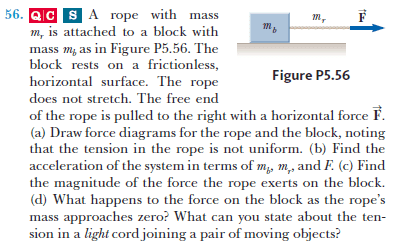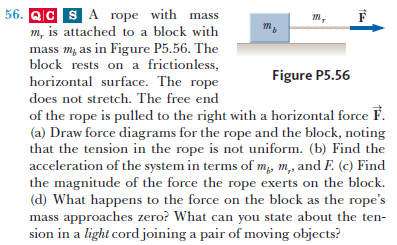# Rope with mass pulling a block

## Homework Statementf = ma

## The Attempt at a Solution

I need help with all parts of this problem, but here are my comments on part a and c:

a) the block has its normal force vector pointing up and its weight vector pointing down. the tension of the rope is pulling it to the right. i'm very confused about the rope's free body diagram.

c) Why should the magnitude of the force that the rope exerts on the block be any different from the magnitude of the force pulling the rope to the right?

PhanthomJay
Science Advisor
Homework Helper
Gold Member

## Homework Statementf = ma

## The Attempt at a Solution

I need help with all parts of this problem, but here are my comments on part a and c:

a) the block has its normal force vector pointing up and its weight vector pointing down. the tension of the rope is pulling it to the right.
Yes , but the rope tension is variable. When drawing a free body diagram of the block alone, the tension force is the rope tension at the connection to the block.
i'm very confused about the rope's free body diagram.
which is not the same as the block's free body diagram. The rope has mass. Isolate the full length of rope. F - Tmin = m_r(a)
c) Why should the magnitude of the force that the rope exerts on the block be any different from the magnitude of the force pulling the rope to the right?
Because in this problem, the rope has mass . The rope tension is F only at the pulling end. It is smaller at the other end where it attaches to the block.
For starters, answer part b first, looking at the rope/block system.

Last edited:
PeterO
Homework Helper

## Homework Statementf = ma

## The Attempt at a Solution

I need help with all parts of this problem, but here are my comments on part a and c:

a) the block has its normal force vector pointing up and its weight vector pointing down. the tension of the rope is pulling it to the right. i'm very confused about the rope's free body diagram.

c) Why should the magnitude of the force that the rope exerts on the block be any different from the magnitude of the force pulling the rope to the right?

You are probably more familiar with problems using a massless [light] string.

To get a feel for this situation, you might like to replace the heavy string, with a light one BUT with a mass added in the middle of the string. - pulling two masses tied together if you like.